Goseeko blog# How to solve LPP by graphical method?

Linear programming problems(LPP) having two variables can be easily solved by a graphical method which provides a pictorial representation of the solution and we get insights into the basic concept used in solve LPP.

## Step by step procedure to solve LPP graphically

1.     First we need to formulate the given problem as a LPP in mathematical form.

2.     Now plot the given constraints on x2 – x1 coordinate plane and find the convex region.

3.     Now determine the convex region and find the value of the objective function at each vertex. The vertex which gives the max or min value of the objective function gives the optimal solution of the LPP.

Note- The region satisfying all the constraints is called feasible region.

## Solved examples

Example: Solve the following LPP graphically-

Maximize-

Subject to the constraints-

Sol.

Change the given constraints into equations-

The line  x1 + 2×2  = 10 meets the x-axis at the point A(10,0) and meets the y-axis at the point B(0,5). Join A and B to get the graph for the line.

Hence origin (0,0) satisfies the inequality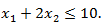so that half plane containing the origin represent the solution set of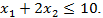AndThe line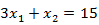meets the x-axis at the point C(5,0) and meets the y-axis at the point D(0,15). Join C and D to get the graph for the line.

Hence origin (0,0) satisfies the inequality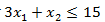so that half plane containing the origin represent the solution set of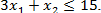Now all the points in first quadrant satisfies

So that first quadrant is the region represented by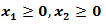Hence the region OCEBO satisfies all the inequalities.

And each point on the region is the feasible solution of the problem.

## Feasible solution

Here in this problem the feasible solution is (5,0), (4,3) and (0,5).

Now we can obtain the optimal solution of the problem.

As we know that any point in the feasible region that gives the optimal value is called an optimal solution.

The value of the objective function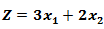at corner points of the feasible region is given below-

Here we see that the maximum value of Z is 18.

## Note

1.    This method is called corner point method-

2.    The optimal value of objective function must occur at a corner point (vertex) of the feasible region

3.    If the feasible region is bounded then the objective function Z has both maximum and minimum values on corner points of the bounded region.

Example: Find the maximum value of Z = 2x + 3y subject to the constraints-

Sol.

Any point (x,y) satisfying the conditions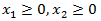lies in the first quadrant only.

Also since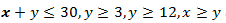and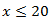the desired point (x,y) lies within the convex region ABCDE.

Its vertices are A(3,3), B(20,3), C(20,10), D(18,12) and E(12,12).

The value of Z at these points are-

Z(A) = 15

Z(B) = 49

Z(C) = 70

Z(D) = 72

Z(E) = 60

Here 72 is the maximum value which is at D.

So that the solution of the LPP is x = 18 and y = 12 and maximum Z  = 72

## Case study

A factory manufactures two types of items, A and B, each type requiring the use of two machines, an automatic and a hand operated. It takes 4 minutes on the automatic and 6 minutes on hand operated machines to manufacture a package of item A, while it takes 6 minutes on automatic and 3 minutes on the hand operated machines to manufacture a package of item B. Each machine is available for at the most 4 hours on any day.

The manufacturer can sell a package of item A at a profit of Rs. 7 and item B at a profit of Rs 10. Assuming that he can sell all the items he manufactures, how many packages of each type should the factory owner produce in a day in order to maximise his profit ? Determine the maximum profit.

Sol.

We can tabulate the data as follows-

Decision variables- Let the manufacturer produce x packages of item A and y packages of item B per day respectively.

Objective function-  Suppose Z denotes the maximum profit,

Z = 7x + 10y

Constraints-

1st constraint-

2nd constraint-

3rd constraint-

## Mathematical formulation

The mathematical form of this LPP can be written as-

Maximize

Z = 7x + 10y

Subject to the constraints-

Tables for the region represented by inequalities-

For the first constraint-

For second condition-

Now we draw on x and y axes.

The coordinates of the vertices O, C, E and B of the feasible region OCEBO are O (0, 0), C (40, 0), E (30, 20) and B (0, 40). The coordinates of E are obtained by solving the equations 4x + 6y = 240 and 6x + 3y = 240.

Therefore Z is maximum at x = 30 and y = 20 and the maximum value of Z is 410.

Interested in learning about similar topics? Here are a few hand-picked blogs for you!